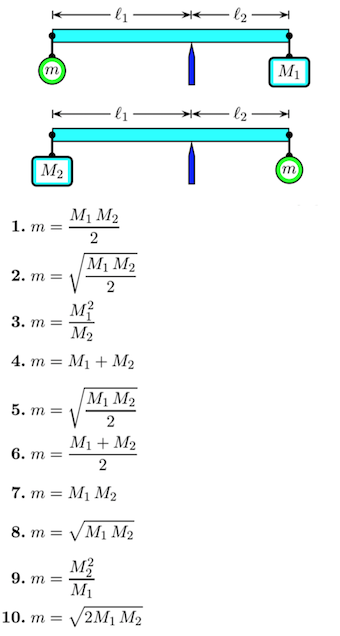# Problem: A rod of negligible mass is pivoted at a point that is off-center, so that length l1 is different from length l2. The figures show two cases in which masses are suspended from the ends of the rod. In each case the unknown mass m is balanced by a known mass M1 or M2 so that the rod remains horizontal.What is the value of m in terms of the known masses?

###### FREE Expert Solution

Net torque for a system in rotational equilibrium:

Στ = 0

Torque:

τ = rFsinθ

Summing the torques on the case with M1:

Take counterclockwise as positive.

81% (50 ratings)###### Problem Details

A rod of negligible mass is pivoted at a point that is off-center, so that length l1 is different from length l2. The figures show two cases in which masses are suspended from the ends of the rod. In each case the unknown mass m is balanced by a known mass M1 or M2 so that the rod remains horizontal.

What is the value of m in terms of the known masses?